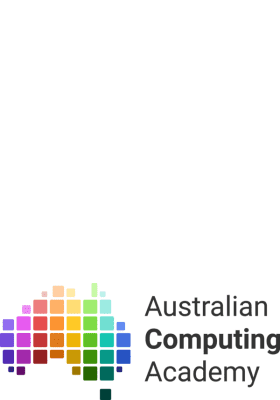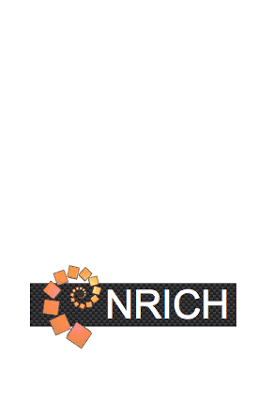# Resources for Year 6 students

## Resources for Year 6 students

Resources are available across all year levels and learning areas, to give children and young people the best opportunity to continue to learn at home.

## Resources

### Resources

•### Measurement and Geometry - Construction of prisms

•### Measurement and Geometry - Construction of prisms and pyramids

•### Measurement and Geometry - Construction of pyramids

•### Measurement and Geometry - Units for measuring capacity

•• ### Number and Algebra - Addition and subtractions of fractions

•### Number and Algebra - Comparing fractions with related denominators

•### Number and Algebra - Connecting fractions and decimals

•### Number and Algebra - Connecting percentages and decimals

•### Number and Algebra - Decimals - Add and subtract

•### Number and Algebra - Decimals - Multiply and divide

•### Number and Algebra - Decimals - Multiply and divide decimals by powers of 10

•### Number and Algebra - Fraction of a quantity

•### Number and Algebra - Percentage discounts

•### Number and Algebra - The order of operations using brackets

•### Number and Algebra - Whole number addition and subtraction

•### Number and Algebra - Whole number multiplication and division

•### Number Guessing with Algorithms

Play a number guessing game, then think about the best way to play the game to win. Create a set of instructions, anyone can follow, then create an algorithm and use it in a harder version of the game.

•### Oh! Harry!

A problem solving task investigating the measurement of cylinders using metric units

•### Olympic starters

Mathematical investigations involving measurement using a stimulus question relating to the Olympics

— 20 Items per Page
Showing 41 - 60 of 95 results.